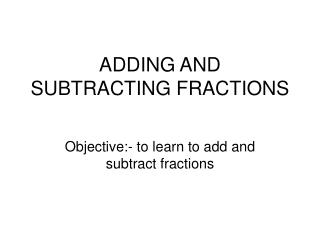DownloadDownload PresentationTélécharger la présentation- - - - - - - - - - - - - - - - - - - - - - - - - - - E N D - - - - - - - - - - - - - - - - - - - - - - - - - - -
##### Presentation Transcript

1. ADDING AND SUBTRACTING FRACTIONS Objective:- to learn to add and subtract fractions

2. Num/Den Numerator REMEMBER: Denominator Addition/Subtraction of Fractions

3. Same Den

4. Adding and subtracting simple fractions 7 – 3 4 – 8 8 3 1 7 2 8 8 1 = = = 2 We can show this calculation in a diagram: – =

5. 9 18 4 7 14 2 - - + = + = = = 11 16 11 16 11 16 11 15 5 2 3 4 19 19 19 7 7 7 Adding / Subtracting Fractions • Adding / Subtracting with same denominator • Only Add / Subtract the numerators, the denominators stay the same.

6. Adding and subtracting simple fractions with different denominators 1 1 3 4 4 4 1 1 2 2 What is + ? + = + =

7. Adding and subtracting simple fractions 3 3 1 8 8 8 1 1 2 2 What is – ? – = – =

8. + Equivalent Multiples of 3 and 4 Equivalent 3 4 6 8 9 12 12 16 15 20 12 is the LCM + Diagram 1 + =

9. - Diagram 1 - Equivalent Multiples of 3 and 4 Equivalent 3 4 6 8 9 12 12 16 15 20 12 is the LCM - =

10. + Diagram 3 + Equivalent Multiples of 4 and 6 Equivalent 4 6 8 12 12 18 16 24 20 30 12 is the LCM + =

11. - Equivalent Multiples of 3 and 4 Equivalent 3 4 6 8 9 12 12 16 15 20 12 is the LCM - =

12. -Diagram 3 - Equivalent Multiples of 4 and 6 Equivalent 4 6 8 12 12 18 16 24 20 30 12 is the LCM - =